# Matplotlib.pyplot.streamplot() in Python

Stream plot is basically a type of 2D plot used majorly by physicists to show fluid flow and 2D field gradients .The basic function to create a stream plot in Matplotlib is:

`ax.streamplot(x_grid, y_grid, x_vec, y_vec, density=spacing)`

Here x_grid and y_grid are arrays of the x and y points.The x_vec and y_vec represent the stream velocity of each point present on the grid.The attribute #density=spacing# specify that how much close the streamlines are to be drawn together.

### Creating stream plot –

Let’s start by creating a simple stream plot that contains streamlines on a 10 by 10 grid.All the streamlines are parallel and pointing towards the right.The code below creates the stream plot containing horizontal parallel lines pointing to the right:

## Python3

 `# Import libraries` `import` `numpy as np` `import` `matplotlib.pyplot as plt`   `# Creating dataset` `x ``=` `np.arange(``0``, ``10``)` `y ``=` `np.arange(``0``, ``10``)`   `# Creating grids` `X, Y ``=` `np.meshgrid(x, y)`   `# x-component to the right` `u ``=` `np.ones((``10``, ``10``)) `   `# y-component zero` `v ``=` `np.zeros((``10``, ``10``)) `   `fig ``=` `plt.figure(figsize ``=` `(``12``, ``7``))`   `# Plotting stream plot` `plt.streamplot(X, Y, u, v, density ``=` `0.5``)`   `# show plot` `plt.show()`

Output: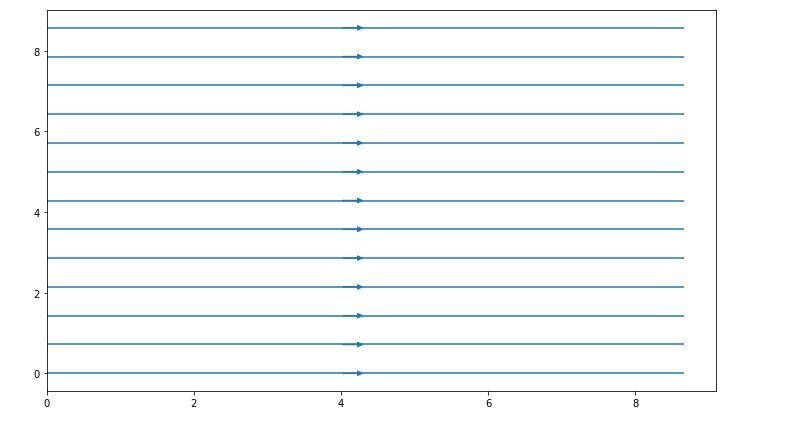Here, x and y are 1D arrays on an evenly spaced grid, u and v are 2D arrays of velocities of x and y where the number of rows should match with the length of y while the number of columns should match with x, density is a float value which controls the closeness of the stream lines.

### Customization of stream plot –

With the help of streamplot() function we can create and customize a plot showing field lines based on defined 2D vector field. Many attributes are available in streamplot() function for the modification of the plots.

## Python3

 `# Import libraries` `import` `numpy as np` `import` `matplotlib.pyplot as plt`   `# Creating data set` `w ``=` `3` `Y, X ``=` `np.mgrid[``-``w:w:``100j``, ``-``w:w:``100j``]` `U ``=` `-``1` `-` `X``*``*``2` `+` `Y` `V ``=` `1` `+` `X ``-` `Y``*``*``2` `speed ``=` `np.sqrt(U``*``*``2` `+` `V``*``*``2``)`   `# Creating plot` `fig ``=` `plt.figure(figsize ``=` `(``12``, ``7``))` `plt.streamplot(X, Y, U, V, density ``=` `1``)`   `# show plot` `plt.show()`

Output: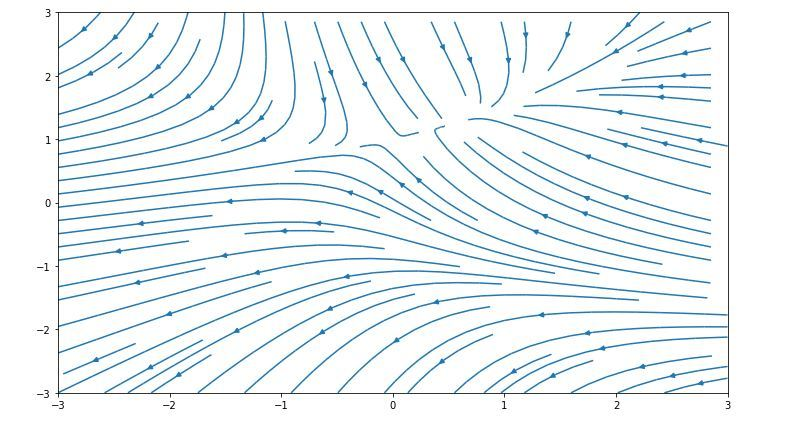Some of the customization of the above graph are listed below:
Varying the density of streamlines –

## Python3

 `import` `numpy as np` `import` `matplotlib.pyplot as plt` `import` `matplotlib.gridspec as gridspec`   `# Creating dataset` `w ``=` `3` `Y, X ``=` `np.mgrid[``-``w:w:``100j``, ``-``w:w:``100j``]` `U ``=` `-``1` `-` `X``*``*``2` `+` `Y` `V ``=` `1` `+` `X ``-` `Y``*``*``2` `speed ``=` `np.sqrt(U``*``*``2` `+` `V``*``*``2``)`   `fig ``=` `plt.figure(figsize ``=``(``24``, ``20``))` `gs ``=` `gridspec.GridSpec(nrows ``=` `3``, ncols ``=` `2``,` `                       ``height_ratios ``=``[``1``, ``1``, ``2``])`   `# Varying the density along a ` `# streamline` `ax ``=` `fig.add_subplot(gs[``0``, ``0``])` `ax.streamplot(X, Y, U, V, ` `              ``density ``=``[``0.4``, ``0.8``])`   `ax.set_title(``'Varying the density along a streamline'``)`   `# show plot` `plt.tight_layout()` `plt.show()`

Output: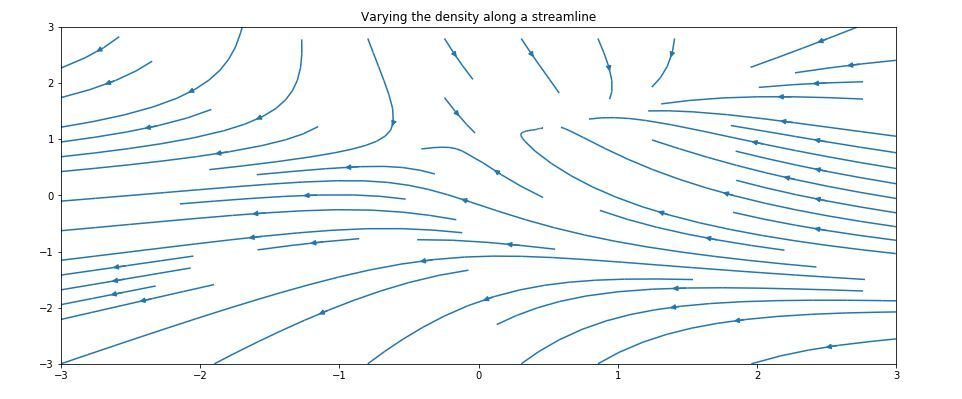Varying the color along a streamline –

## Python3

 `import` `numpy as np` `import` `matplotlib.pyplot as plt` `import` `matplotlib.gridspec as gridspec`   `# Creating dataset` `w ``=` `3` `Y, X ``=` `np.mgrid[``-``w:w:``100j``, ``-``w:w:``100j``]` `U ``=` `-``1` `-` `X``*``*``2` `+` `Y` `V ``=` `1` `+` `X ``-` `Y``*``*``2` `speed ``=` `np.sqrt(U``*``*``2` `+` `V``*``*``2``)`   `fig ``=` `plt.figure(figsize ``=``(``24``, ``20``))` `gs ``=` `gridspec.GridSpec(nrows ``=` `3``, ncols ``=` `2``,` `                       ``height_ratios ``=``[``1``, ``1``, ``2``])`   `# Varying color along a streamline` `ax ``=` `fig.add_subplot(gs[``0``, ``1``])` `strm ``=` `ax.streamplot(X, Y, U, V, color ``=` `U,` `                     ``linewidth ``=` `2``, cmap ``=``'autumn'``)` `fig.colorbar(strm.lines)` `ax.set_title(``'Varying the color along a streamline.'``)`   `# show plot` `plt.tight_layout()` `plt.show()    `

Output: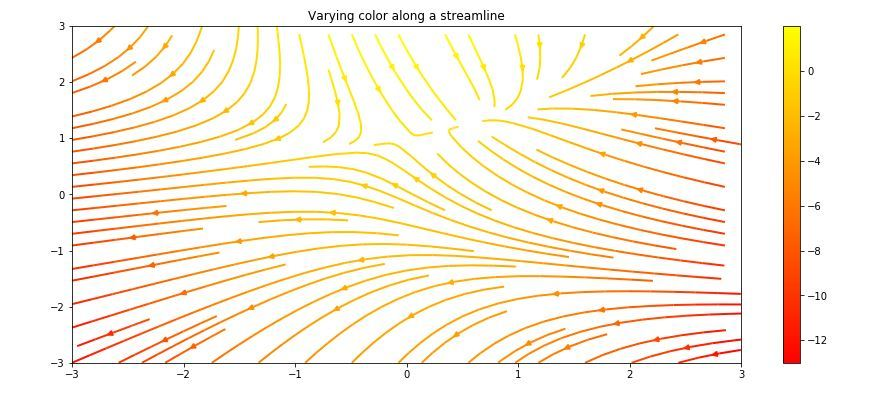Varying the line width along a streamline –

## Python3

 `import` `numpy as np` `import` `matplotlib.pyplot as plt` `import` `matplotlib.gridspec as gridspec`   `# Creating dataset` `w ``=` `3` `Y, X ``=` `np.mgrid[``-``w:w:``100j``, ``-``w:w:``100j``]` `U ``=` `-``1` `-` `X``*``*``2` `+` `Y` `V ``=` `1` `+` `X ``-` `Y``*``*``2` `speed ``=` `np.sqrt(U``*``*``2` `+` `V``*``*``2``)`   `fig ``=` `plt.figure(figsize ``=``(``24``, ``20``))` `gs ``=` `gridspec.GridSpec(nrows ``=` `3``, ncols ``=` `2``, ` `                       ``height_ratios ``=``[``1``, ``1``, ``2``])`   `# Varying line width along a streamline` `ax ``=` `fig.add_subplot(gs[``1``, ``0``])` `lw ``=` `5` `*` `speed ``/` `speed.``max``()` `ax.streamplot(X, Y, U, V, density ``=` `0.6``, ` `              ``color ``=``'k'``, linewidth ``=` `lw)`   `ax.set_title(``'Varying line width along a streamline'``)`   `# show plot` `plt.tight_layout()` `plt.show()`

Output: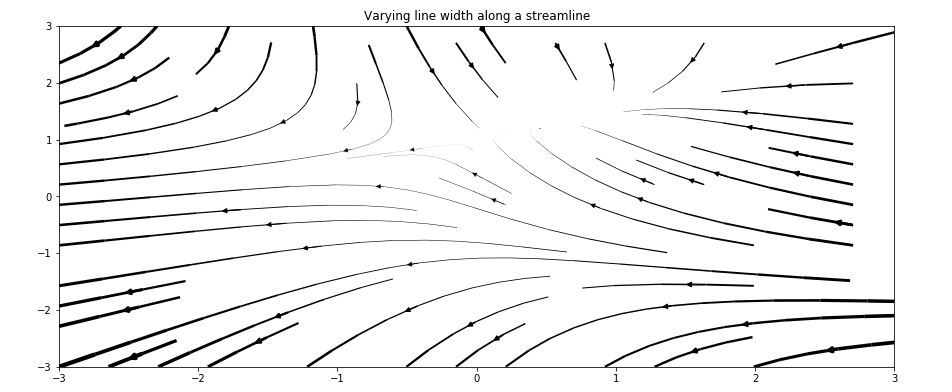Controlling the starting points of streamlines –

## Python3

 `import` `numpy as np` `import` `matplotlib.pyplot as plt` `import` `matplotlib.gridspec as gridspec`   `# Creating dataset` `w ``=` `3` `Y, X ``=` `np.mgrid[``-``w:w:``100j``, ``-``w:w:``100j``]` `U ``=` `-``1` `-` `X``*``*``2` `+` `Y` `V ``=` `1` `+` `X ``-` `Y``*``*``2` `speed ``=` `np.sqrt(U``*``*``2` `+` `V``*``*``2``)`   `fig ``=` `plt.figure(figsize ``=``(``24``, ``20``))` `gs ``=` `gridspec.GridSpec(nrows ``=` `3``, ncols ``=` `2``,` `                       ``height_ratios ``=``[``1``, ``1``, ``2``])`   `# Controlling the starting points` `# of the streamlines` `seek_points ``=` `np.array([[``-``2``, ``-``1``, ``0``, ``1``, ``2``, ``-``1``],` `                        ``[``-``2``, ``-``1``,  ``0``, ``1``, ``2``, ``2``]])`   `ax ``=` `fig.add_subplot(gs[``1``, ``1``])` `strm ``=` `ax.streamplot(X, Y, U, V, color ``=` `U, ` `                     ``linewidth ``=` `2``,` `                     ``cmap ``=``'autumn'``,` `                     ``start_points ``=` `seek_points.T)`   `fig.colorbar(strm.lines)` `ax.set_title('Controlling the starting\` `points of the streamlines')`   `# Displaying the starting points` `# with blue symbols.` `ax.plot(seek_points[``0``], seek_points[``1``], ``'bo'``)` `ax.``set``(xlim ``=``(``-``w, w), ylim ``=``(``-``w, w))`   `# show plot` `plt.tight_layout()` `plt.show()`

Output: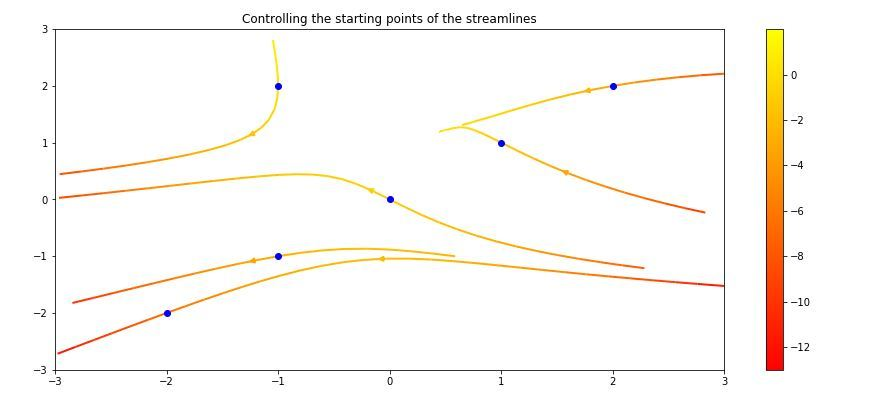Streamlines skipping masked regions and NaN values –

## Python3

 `# Import libraries` `import` `numpy as np` `import` `matplotlib.pyplot as plt` `import` `matplotlib.gridspec as gridspec`   `# Creating dataset` `w ``=` `3` `Y, X ``=` `np.mgrid[``-``w:w:``100j``, ``-``w:w:``100j``]` `U ``=` `-``1` `-` `X``*``*``2` `+` `Y` `V ``=` `1` `+` `X ``-` `Y``*``*``2` `speed ``=` `np.sqrt(U``*``*``2` `+` `V``*``*``2``)`   `fig ``=` `plt.figure(figsize ``=``(``20``, ``16``))` `gs ``=` `gridspec.GridSpec(nrows ``=` `3``, ncols ``=` `2``, height_ratios ``=``[``1``, ``1``, ``2``])`   `# Create a mask` `mask ``=` `np.zeros(U.shape, dtype ``=` `bool``)` `mask[``40``:``60``, ``40``:``60``] ``=` `True` `U[:``20``, :``20``] ``=` `np.nan` `U ``=` `np.ma.array(U, mask ``=` `mask)`   `ax ``=` `fig.add_subplot(gs[``2``:, :])` `ax.streamplot(X, Y, U, V, color ``=``'r'``)` `ax.set_title(``'Streamplot with Masking'``)`   `ax.imshow(~mask, extent ``=``(``-``w, w, ``-``w, w), alpha ``=` `0.5``,` `          ``interpolation ``=``'nearest'``, cmap ``=``'gray'``, aspect ``=``'auto'``)` `ax.set_aspect(``'equal'``)`   `# show plot` `plt.tight_layout()` `plt.show()`

Output: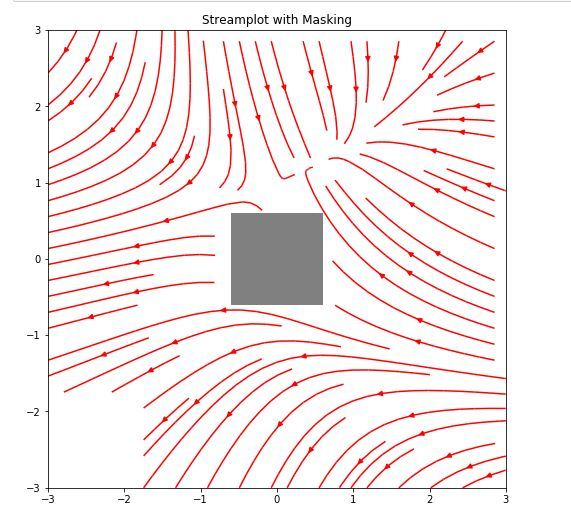Example:
Stream plot to demonstrate the electric field due to two point charges.The electric field at any point on a surface depends upon the position and distance between the two charges:

## Python3

 `import` `sys` `import` `numpy as np` `import` `matplotlib.pyplot as plt` `from` `matplotlib.patches ``import` `Circle`   `# Function to determine electric field` `def` `E(q, r0, x, y):` `    ``den ``=` `np.hypot(x``-``r0[``0``], y``-``r0[``1``])``*``*``3` `    ``return` `q ``*` `(x ``-` `r0[``0``]) ``/` `den, q ``*` `(y ``-` `r0[``1``]) ``/` `den`   `# Grid of x, y points` `nx, ny ``=` `64``, ``64` `x ``=` `np.linspace(``-``2``, ``2``, nx)` `y ``=` `np.linspace(``-``2``, ``2``, ny)` `X, Y ``=` `np.meshgrid(x, y)`   `# Create a multipole with nq charges of` `# alternating sign, equally spaced` `# on the unit circle.`   `# Increase the power with increase in charge` `nq ``=` `2``*``*``1` `charges ``=` `[]` `for` `i ``in` `range``(nq):` `    ``q ``=` `i ``%` `2` `*` `2` `-` `1` `    ``charges.append((q, (np.cos(``2` `*` `np.pi ``*` `i ``/` `nq),` `                        ``np.sin(``2` `*` `np.pi ``*` `i ``/` `nq))))`   `# Electric field vector, E =(Ex, Ey)` `# as separate components` `Ex, Ey ``=` `np.zeros((ny, nx)), np.zeros((ny, nx))`   `for` `charge ``in` `charges:` `    ``ex, ey ``=` `E(``*``charge, x ``=` `X, y ``=` `Y)` `    ``Ex ``+``=` `ex` `    ``Ey ``+``=` `ey`   `fig ``=` `plt.figure(figsize ``=``(``18``, ``8``))` `ax ``=` `fig.add_subplot(``111``)`   `# Plotting the streamlines with ` `# proper color and arrow` `color ``=` `2` `*` `np.log(np.hypot(Ex, Ey))` `ax.streamplot(x, y, Ex, Ey, color ``=` `color,` `              ``linewidth ``=` `1``, cmap ``=` `plt.cm.inferno,` `              ``density ``=` `2``, arrowstyle ``=``'->'``, ` `              ``arrowsize ``=` `1.5``)`   `# Add filled circles for the charges` `# themselves` `charge_colors ``=` `{``True``: ``'#AA0000'``, ` `                 ``False``: ``'#0000AA'``}`   `for` `q, pos ``in` `charges:` `    ``ax.add_artist(Circle(pos, ``0.05``, ` `                         ``color ``=` `charge_colors[q>``0``]))`   `ax.set_xlabel(``'X-axis'``)` `ax.set_ylabel(``'X-axis'``)` `ax.set_xlim(``-``2``, ``2``)` `ax.set_ylim(``-``2``, ``2``)` `ax.set_aspect(``'equal'``)`   `plt.show()`

Output: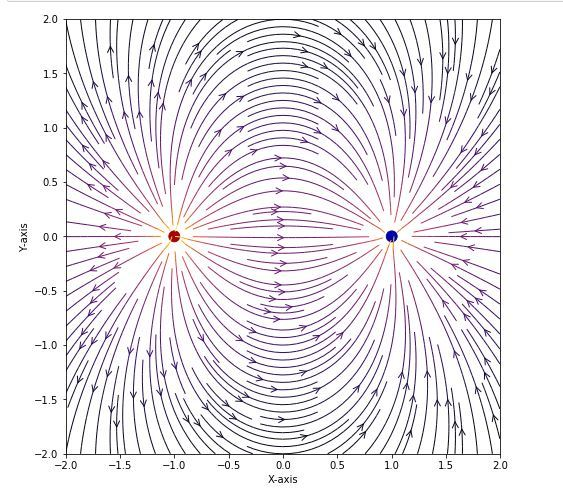Whether you're preparing for your first job interview or aiming to upskill in this ever-evolving tech landscape, GeeksforGeeks Courses are your key to success. We provide top-quality content at affordable prices, all geared towards accelerating your growth in a time-bound manner. Join the millions we've already empowered, and we're here to do the same for you. Don't miss out - check it out now!

Previous
Next